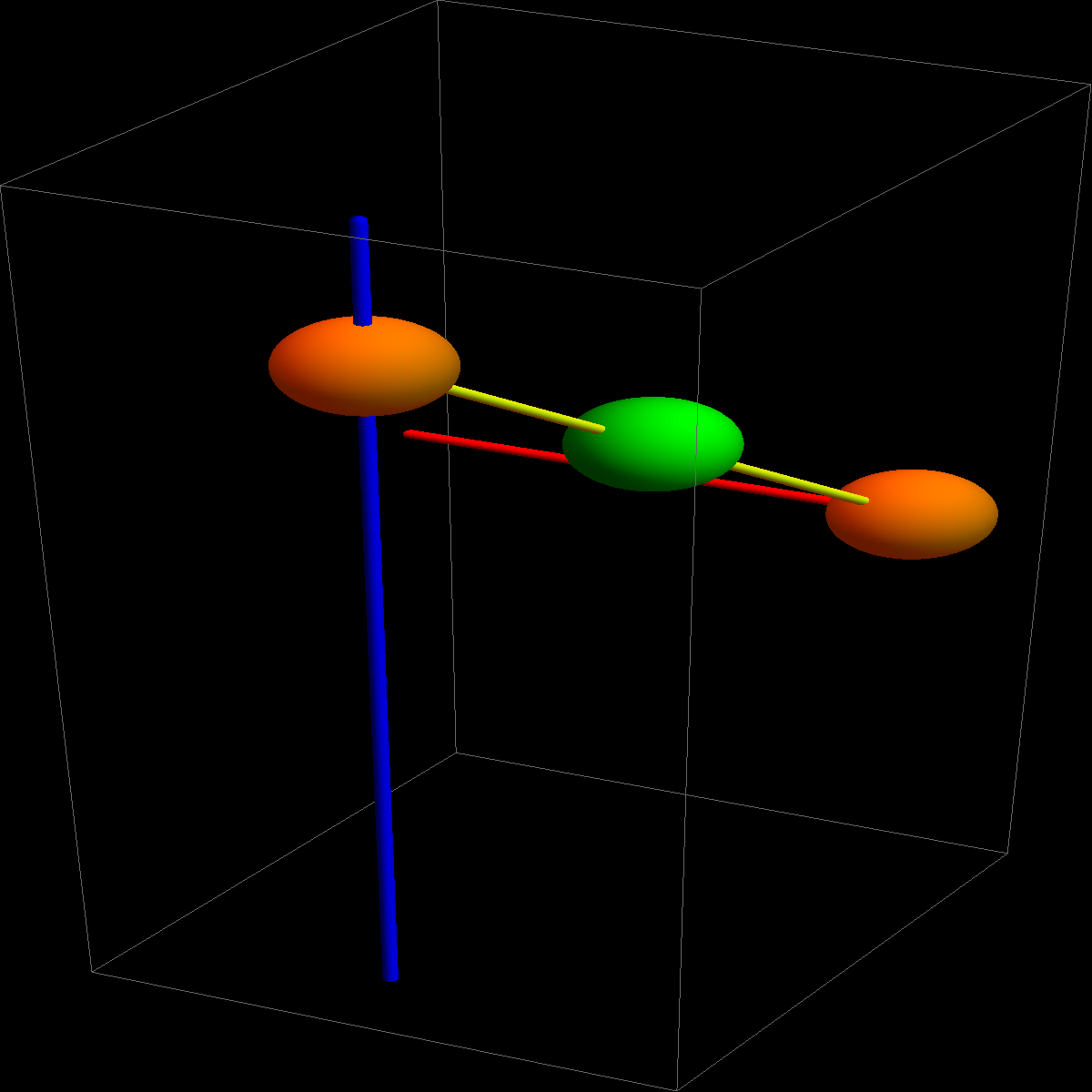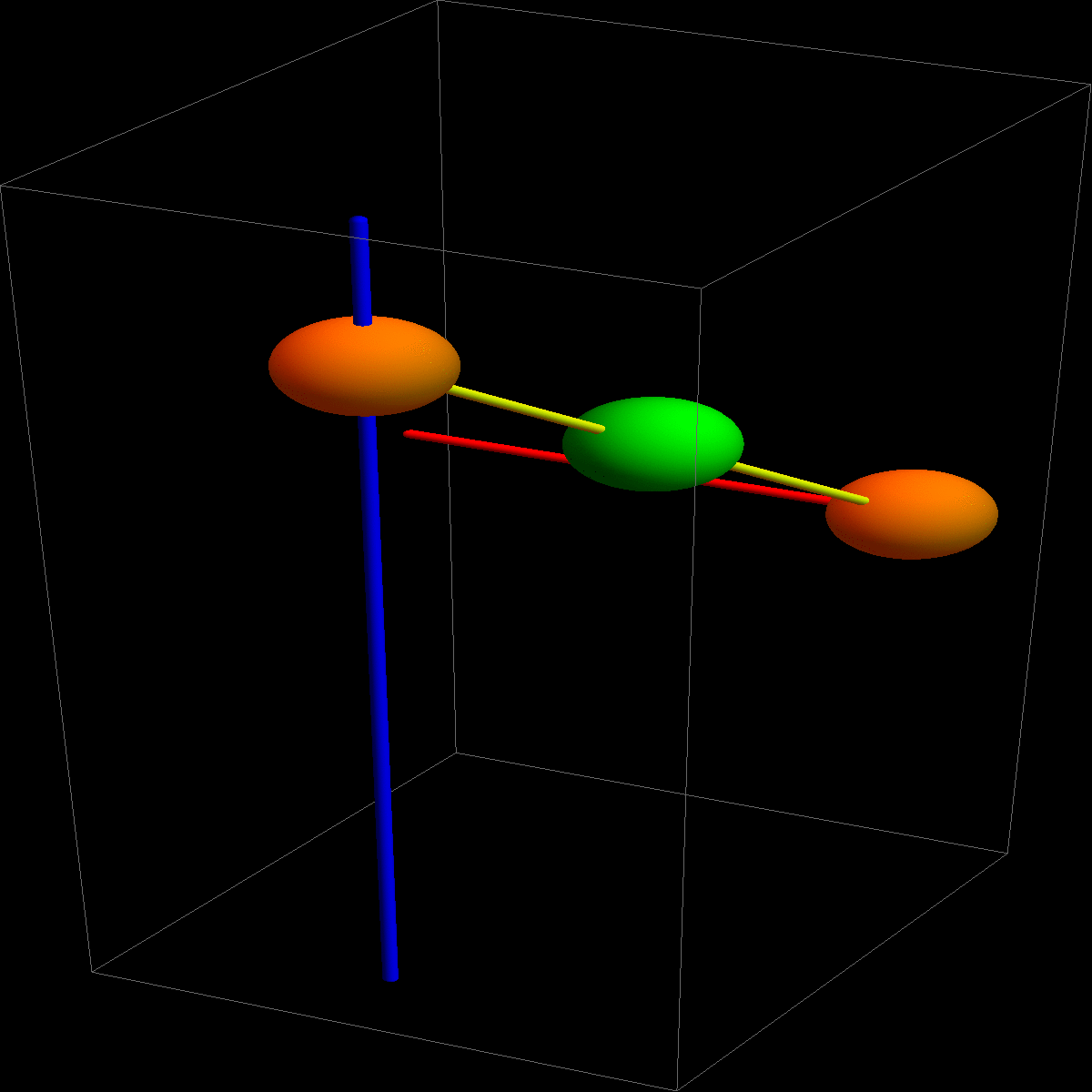## TF 20 of First hourly

It is an old Harvard tradition to ask conceptional true-false questions, also more difficult ones. We know that they are difficult but they are of great value when preparing for exams. Yes, not all students like these questions as they can be uncomfortable. It is always uncomfortable to get out of the comfort zone! TF problem 20 asked:

 Given two lines r(t) = P+tv and s(t) = Q+tw. Is the point (P+Q)/2 equidistant to the lines?The answer is NO. Below is an illustration which hopefully makes it clear. We knew that the problem is difficult. (Oliver wrote it and got it wrong himself at first). One can learn a lot from it:
• In order to analyze a question, it is good to look at extreme cases. This is a general principle when probing questions, in any science, in any field. Extreme cases like when one point is very far away and the other point is nearby immediately tell that it is wrong.
• It is also not always the case that an immediate argument how convincing it is, is correct. Oliver (and he was not alone) argued like that: the midpoint M is on the plane equidistant to the two lines. Ergo, the point M is equidistant to the two lines. It sounds convincing. But it is not true.
• A small experiment with two pens can show why the result is false. It would actually have been possible to do the experiment quickly while writing the exam. We know that the optimal time spent for one TF question is 30 seconds, but it would be possible to do the experiment in that time.
Here is citation from a letter from September 7 of Dean Michael Smith of FAS about the new Gen Ed 2.0: "Gen Ed courses should take what's familiar and de-familiarize it. In particular, the courses should unsettle our students' ingrained assumptions. "
The True/False problem 20 is unsettling. It illustrates that things which appear to be obvious are not so obvious. We don't talk about difficult ethical or political questions in math 21a, but about the most pure science there is and about one of the most concrete situations we can imagine: two lines in space.

By the way, the art of asking good question is cultivated in many mathematics departments. One of the researchers who has spear headed this since decades is Maria Terrell from Cornell. You find a list of Good questions on her website.In case you want to look at it in Mathematica, here is the code:
```(* TF Problem 20 of Midterm 1, Harvard Math 21a, Fall 2017 *)
Manipulate[
S1 = Graphics3D[{Red, Tube[{{-1, 2, 0}, {M, 2, 0}}]}];
S2 = Graphics3D[{Blue, Tube[{{0, 0, -3}, {0, 0, 3}}]}];
S3 = Graphics3D[{Orange, Sphere[{0, 0, 2}, 0.3]}];
S4 = Graphics3D[{Orange, Sphere[{M, 2, 0}, 0.3]}];
S5 = Graphics3D[{Black, Tube[{{0, 0, 2}, {M, 2, 0}}]}];
S6 = Graphics3D[{Green, Sphere[{M/2, 1, 1}, 0.3]}];
Show[{S1, S2, S3, S4, S5, S6}], {M, 1, 30}]
```